# NCERT Solutions for Class 11 Physics chapter 11 - Thermal Properties of Matter - Free PDF download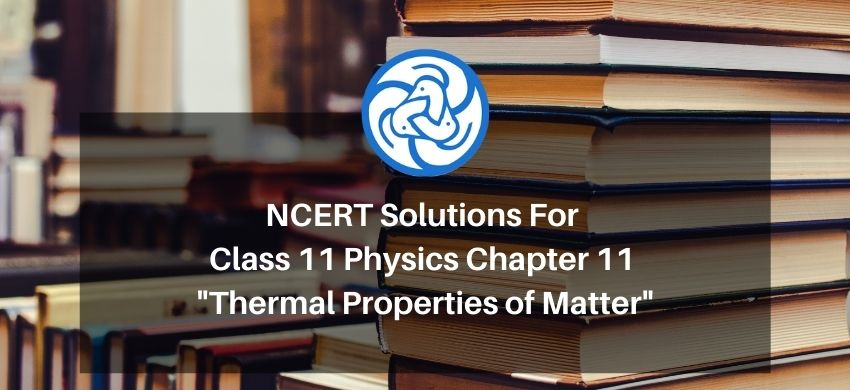Hey, are you a class 11 student and looking for ways to download NCERT Solutions for Class 11 Physics chapter 11 "Thermal Properties of Matter"? If yes. Then read this post till the end.

In this article, we have listed NCERT solution for class 11 Physics Chapter 11 in PDF that are prepared by Kota’s top IITian’s Faculties by keeping Simplicity in mind.

If you want to learn and understand class 11 Physics chapter 11 "Thermal Properties of Matter" in an easy way then you can use these solutions PDF.

NCERT Solutions helps students to Practice important concepts of subjects easily. Class 11 Physics solutions provide detailed explanations of all the NCERT questions that students can use to clear their doubts instantly.

If you want to score high in your class 11 Physics Exam then it is very important for you to have a good knowledge of all the important topics, so to learn and practice those topics you can use eSaral NCERT Solutions.

So, without wasting more time Let’s start.

### Download The PDF of NCERT Solutions for Class 11 Physics Chapter 11 "Thermal Properties of Matter"

Question.1: The triple points of neon and carbon dioxide are 24.57 K and 216.55 K respectively. Express these temperatures on the Celsius and Fahrenheit scales.

Solution. Kelvin and Celsius scales are related as:
$T_{\mathrm{C}}=T_{K}-273.15 \ldots(i)$
Celsius and Fahrenheit scales are related as:
$T_{\mathrm{F}}=\frac{9}{5} T_{\mathrm{C}}+32$$\ldots(i i) For neon: T_{K}=24.57 \mathrm{~K} \therefore T_{\mathrm{C}}=24.57-273.15=-248.58^{\circ} \mathrm{C} T_{\mathrm{F}}=\frac{9}{5} T_{\mathrm{C}}+32 =\frac{9}{5}(-248.58)+32 =415.44^{\circ} \mathrm{F} For carbon dioxide: T_{K}=216.55 \mathrm{~K} \therefore T_{\mathrm{C}}=216.55-273.15=-56.60^{\circ} \mathrm{C} T_{\mathrm{F}}=\frac{9}{5}\left(T_{\mathrm{C}}\right)+32 \quad=\frac{9}{5}(-56.60)+32 \quad=-69.88^{\circ} \mathrm{C} Question 2: Two absolute scales A and B have triple points of water defined to be 200 A and 350 B. What is the relation between T_{A} and T_{B}? Solution. Triple point of water on absolute scaleA, T_{1}=200 \mathrm{~A} Triple point of water on absolute scale \mathrm{B}, T_{2}=350 \mathrm{~B} Triple point of water on Kelvin scale, T_{K}=273.15 \mathrm{~K} The temperature 273.15 \mathrm{~K} on Kelvin scale is equivalent to 200 \mathrm{~A} on absolute scale \mathrm{A}. T_{1}=T_{K} 200 \mathrm{~A}=273.15 \mathrm{~K} \therefore \mathrm{A}=\frac{273.15}{200} The temperature 273.15 K on Kelvin scale is equivalent to 350 B on absolute scale B. T_{2}=T_{K} 350 \mathrm{~B}=273.15 \therefore \mathrm{B}=\frac{273.15}{350} T_{A} is triple point of water on scale A. T_{B} is triple point of water on scale B. \therefore \frac{273.15}{200} \times T_{\mathrm{A}}=\frac{273.15}{350} \times T_{\mathrm{B}} T_{\mathrm{A}}=\frac{200}{350} T_{\mathrm{B}} Therefore, the ratio T_{\mathrm{A}}: T_{\mathrm{B}} is given as 4: 7. Question.3: The electrical resistance in ohms of a certain thermometer varies with temperature according to the approximate law: R=R_{0}\left[1+a\left(T-T_{0}\right)\right] The resistance is 101.6 \Omega at the triple-point of water 273.16 \mathrm{~K}, and 165.5 \Omega at the normal melting point of lead (600.5 \mathrm{~K}). What is the temperature when the resistance is 123.4 \Omega ? Solution. It is given that: R=R_{0}\left[1+\alpha\left(T-T_{0}\right)\right] \ldots(i) Where, R_{0} and T_{0} are the initial resistance and temperature respectively R and T are the final resistance and temperature respectively a is a constant At the triple point of water, T_{0}=273.15 \mathrm{~K} Resistance of lead, R_{0}=101.6 \Omega At normal melting point of lead, T=600.5 \mathrm{~K} Resistance of lead, R=165.5 \Omega Substituting these values in equation (i), we get: R=R_{0}\left[1+\alpha\left(T-T_{0}\right)\right] 165.5=101.6[1+\alpha(600.5-273.15)] 1.629=1+\alpha(327.35) \therefore \alpha=\frac{0.629}{327.35}=1.92 \times 10^{-3} \mathrm{~K}^{-1} For resistance, R_{1}=123.4 \Omega \mathrm{R}_{1}=\mathrm{R}_{0}\left[1+\alpha\left(\mathrm{T}-\mathrm{T}_{0}\right)\right] Where, \mathrm{T} is the temperature when the resis tan ce of lead is 123.4 \Omega 123.4=101.6\left[1+1.92 \times 10^{-3}(\mathrm{~T}-273.15)\right] 1.214=1+1.92 \times 10^{-3}(\mathrm{~T}-273.15) \frac{0.214}{1.92 \times 10^{-3}}=\mathrm{T}-273.15 \therefore \mathrm{T}=384.61 \mathrm{~K} Question.4: Answer the following: (a) The triple-point of water is a standard fixed point in modern thermometry. Why? What is wrong in taking the melting point of ice and the boiling point of water as standard fixed points (as was originally done in the Celsius scale)? (b) There were two fixed points in the original Celsius scale as mentioned above which were assigned the number 0^{\circ} \mathrm{C} and 100^{\circ} \mathrm{C} respectively. On the absolute scale, one of the fixed points is the triple-point of water, which on the Kelvin absolute scale is assigned the number 273.16 \mathrm{~K}. What is the other fixed point on this (Kelvin) scale? (c) The absolute temperature (Kelvin scale) T is related to the temperature t_{\mathrm{c}} on the Celsius scale by t_{\mathrm{c}}=T-273.15 Why do we have 273.15 in this relation, and not 273.16? (d) What is the temperature of the triple-point of water on an absolute scale whose unit interval size is equal to that of the Fahrenheit scale? Solution. (a) The triple point of water has a unique value of 273.16 \mathrm{~K}. At particular values of volume and pressure, the triple point of water is always 273.16 \mathrm{~K}. The melting point of ice and boiling point of water do not have particular values because these points depend on pressure and temperature. (b) The absolute zero or 0 \mathrm{~K} is the other fixed point on the Kelvin absolute scale. (c) The temperature 273.16 \mathrm{~K} is the triple point of water. It is not the melting point of ice. The temperature 0^{\circ} \mathrm{C} on Celsius scale is the melting point of ice. Its corresponding value on Kelvin scale is 273.15 \mathrm{~K}. Hence, absolute temperature (Kelvin scale) T, is related to temperature t_{\mathrm{c}}, on Celsius scale as: t_{\mathrm{c}}=T-273.15 (d) Let T_{F} be the temperature on Fahrenheit scale and T_{K} be the temperature on absolute scale. Both the temperatures can be related as: \frac{T_{\mathrm{F}}-32}{180}=\frac{T_{\mathrm{K}}-273.15}{100} \quad \ldots$$(i)$
Let $T_{F 1}$ be the temperature on Fahrenheit scale and $T_{K 1}$ be the temperature on absolute scale. Both the temperatures can be related as
$\frac{T_{\mathrm{Fl}}-32}{180}=\frac{T_{\mathrm{Kl}}-273.15}{100}$$\ldots(i i) It is given that: T_{K 1}-T_{K}=1 \mathrm{~K} Subtracting equation (i) from equation (ii), we get: \frac{T_{\mathrm{Fl}}-T_{\mathrm{F}}}{180}=\frac{T_{\mathrm{Kl}}-T_{\mathrm{K}}}{100}=\frac{1}{100} T_{\mathrm{Fl}}-T_{\mathrm{F}}=\frac{1 \times 180}{100}=\frac{9}{5} Triple point of water =273.16 \mathrm{~K} \therefore Triple point of water on absolute scale =273.16 \times \frac{9}{5}=491.69 Question 5: Two ideal gas thermometers And Buse oxygen and hydrogen respectively. The following observations are made: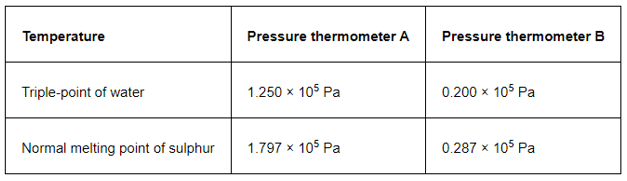(a) What is the absolute temperature of normal melting point of sulphur as read by thermometers Aand B? (b) What do you think is the reason behind the slight difference in answers of thermometers Aand B? (The thermometers are not faulty). What further procedure is needed in the experiment to reduce the discrepancy between the two readings? Solution. (a) Triple point of water, T=273.16 \mathrm{~K}. At this temperature, pressure in thermometer \mathrm{A}, P_{\mathrm{A}}=1.250 \times 10^{5} \mathrm{~Pa} Let T_{1} be the normal melting point of sulphur. At this temperature, pressure in thermometer \mathrm{A}, P_{1}=1.797 \times 10^{5} \mathrm{~Pa} According to Charles' law, we have the relation: \frac{P_{\mathrm{A}}}{T}=\frac{P_{1}}{T_{1}} \therefore T_{1}=\frac{P_{1} T}{P_{\mathrm{A}}}=\frac{1.797 \times 10^{5} \times 273.16}{1.250 \times 10^{5}} =392.69 \mathrm{~K} Therefore, the absolute temperature of the normal melting point of sulphur as read by thermometer \mathrm{A} is 392.69 \mathrm{~K}. At triple point 273.16 \mathrm{~K}, the pressure in thermometer \mathrm{B}, \mathrm{P}_{\mathrm{B}}=0.200 \times 10^{5} \mathrm{~Pa} At temperature T_{1}, the pressure in thermometer \mathrm{B}, \mathrm{P}_{2}=0.287 \times 10^{5} \mathrm{~Pa} According to Charles' law, we can write the relation: \frac{P_{\mathrm{B}}}{T}=\frac{P_{1}}{T_{1}} \frac{0.200 \times 10^{5}}{273.16}=\frac{0.287 \times 10^{5}}{T_{1}} \therefore T_{1}=\frac{0.287 \times 10^{5}}{0.200 \times 10^{5}} \times 273.16=391.98 \mathrm{~K} Therefore, the absolute temperature of the normal melting point of sulphur as read by thermometer \mathrm{B} is 391.98 \mathrm{~K}. (b) The oxygen and hydrogen gas present in thermometers A and B respectively are not perfect ideal gases. Hence, there is a slight difference between the readings of thermometers A and B. To reduce the discrepancy between the two readings, the experiment should be carried under low pressure conditions. At low pressure, these gases behave as perfect ideal gases. Question.6: is 45.0^{\circ} \mathrm{C}. What is the actual length of the steel rod on that day? What is the length of the same steel rod on a day when the temperature is 27.0^{\circ} \mathrm{C} ? Coefficient of linear expansion of steel =1.20 \times 10^{-5} \mathrm{~K}^{-1} Solution. Length of the steel tape at temperature T=27^{\circ} \mathrm{C}, I=1 \mathrm{~m}=100 \mathrm{~cm} At temperature T_{1}=45^{\circ} \mathrm{C}, the length of the steel rod, l_{1}=63 \mathrm{~cm} Coefficient of linear expansion of steel, \alpha=1.20 \times 10^{-5} \mathrm{~K}^{-1} Let I_{2} be the actual length of the steel rod and I be the length of the steel tape at 45^{\circ} \mathrm{C}. l^{\prime}=l+\alpha l\left(T_{1}-T\right) \therefore l^{\prime}=100+1.20 \times 10^{-5} \times 100(45-27) =100.0216 \mathrm{~cm} Hence, the actual length of the steel rod measured by the steel tape at 45^{\circ} \mathrm{C} can be calculated as: l_{2}=\frac{100.0216}{100} \times 63=63.0136 \mathrm{~cm} Therefore, the actual length of the rod at 45.0^{\circ} \mathrm{C} is 63.0136 \mathrm{~cm}. Its length at 27.0^{\circ} \mathrm{C} is 63.0 \mathrm{~cm}. Question.7 A large steel wheel is to be fitted on to a shaft of the same material. At 27^{\circ} \mathrm{C}, the outer diameter of the shaft is 8.70 \mathrm{~cm} and the diameter of the central hole in the wheel is 8.69 \mathrm{~cm}. The shaft is cooled using 'dry ice'. At what temperature of the shaft does the wheel slip on the shaft? Assume coefficient of linear expansion of the steel to be constant over the required temperature range: a_{\text {steel }}=1.20 \times 10^{-5} \mathrm{~K}^{-} Solution. The given temperature, T=27^{\circ} \mathrm{C} can be written in Kelvin as: 27+273=300 \mathrm{~K} Outer diameter of the steel shaft at T, d_{1}=8.70 \mathrm{~cm} Diameter of the central hole in the wheel at T, d_{2}=8.69 \mathrm{~cm} Coefficient of linear expansion of steel, \alpha_{\text {steel }}=1.20 \times 10^{-5} \mathrm{~K}^{-1} After the shaft is cooled using 'dry ice', its temperature becomes T_{1}. The wheel will slip on the shaft, if the change in diameter, \Delta d=8.69-8.70 =-0.01 \mathrm{~cm} Temperature T_{1}, can be calculated from the relation: \Delta d=d_{1} \alpha_{\text {steel }}\left(T_{1}-T\right) 0.01=8.70 \times 1.20 \times 10^{-5}\left(T_{1}-300\right) \left(T_{1}-300\right)=95.78 \therefore T_{1}=204.21 \mathrm{~K} =204.21-273.16 =-68.95^{\circ} \mathrm{C} Therefore, the wheel will slip on the shaft when the temperature of the shaft is –69°C. Question.8:A hole is drilled in a copper sheet. The diameter of the hole is 4.24 \mathrm{~cm} at 27.0^{\circ} \mathrm{C}. What is the change in the diameter of the hole when the sheet is heated to 227^{\circ} \mathrm{C} ? Coefficient of linear expansion of copper =1.70 \times 10^{-5} \mathrm{~K}^{-1}. Solution. Initial temperature, T_{1}=27.0^{\circ} \mathrm{C} Diameter of the hole at T_{1}, d_{1}=4.24 \mathrm{~cm} Final temperature, T_{2}=227^{\circ} \mathrm{C} Diameter of the hole at T_{2}=d_{2} Co-efficient of linear expansion of copper, \alpha_{\mathrm{Cu}}=1.70 \times 10^{-5} \mathrm{~K}^{1} For co-efficient of superficial expansion \beta,and change in temperature \Delta T, we have the relation: \frac{\text { Change in area }(\Delta A)}{\text { Original area }(A)}=\beta \Delta T \frac{\left(\pi \frac{d_{2}^{2}}{4}-\pi \frac{d_{1}^{2}}{4}\right)}{\left(\pi \frac{d_{1}^{2}}{4}\right)}=\frac{\Delta A}{A} \therefore \frac{\Delta A}{A}=\frac{d_{2}^{2}-d_{1}^{2}}{d_{1}^{2}} But \beta=2 \alpha \therefore \frac{d_{2}^{2}-d_{1}^{2}}{d_{1}^{2}}=2 \alpha \Delta T \frac{d_{2}^{2}}{d_{1}^{2}}-1=2 \alpha\left(T_{2}-T_{1}\right) \frac{d_{2}^{2}}{(4.24)^{2}}=2 \times 1.7 \times 10^{-5}(227-27)+1 d_{2}^{2}=17.98 \times 1.0068=18.1 \therefore d_{2}=4.2544 \mathrm{~cm} Change in diameter =d_{2}-d_{1}=4.2544-4.24=0.0144 \mathrm{~cm} Hence, the diameter increases by 1.44 \times 10^{-2} \mathrm{~cm}. Question .9 A brass wire 1.8 \mathrm{~m} long at 27^{\circ} \mathrm{C} is held taut with little tension between two rigid supports. If the wire is cooled to a temperature of -39^{\circ} \mathrm{C}, what is the tension developed in the wire, if its diameter is 2.0 \mathrm{~mm} ? Co-efficient of linear expansion of brass =2.0 \times 10^{-5} \mathrm{~K}^{-1}; Young's modulus of brass =0.91 \times 10^{11} \mathrm{~Pa}. Solution. Initial temperature, T_{1}=27^{\circ} \mathrm{C} Length of the brass wire at T_{1}, I=1.8 \mathrm{~m} Final temperature, T_{2}=-39^{\circ} \mathrm{C} Diameter of the wire, d=2.0 \mathrm{~mm}=2 \times 10^{-3} \mathrm{~m} Tension developed in the wire =F Coefficient of linear expansion of brass, \alpha=2.0 \times 10^{-5} \mathrm{~K}^{-1} Young's modulus of brass, Y=0.91 \times 10^{11} \mathrm{~Pa} Young's modulus is given by the relation: Y=\frac{\text { Stress }}{\text { Strain }}=\frac{\frac{F}{A}}{\frac{\Delta L}{L}} \Delta L=\frac{F \times L}{A \times Y}$$\ldots(i)$
Where,
$F=$ Tension developed in the wire
$A=$ Area of cross-section of the wire.
$\Delta L=$ Change in the length, given by the relation:
$\Delta L=\alpha L\left(T_{2}-T_{1}\right) \ldots(i i)$ Equating equations (i) and (ii), we get:
$\alpha L\left(T_{2}-T_{1}\right)=\frac{F L}{\pi\left(\frac{d}{2}\right)^{2} \times Y}$
$F=\alpha\left(T_{2}-T_{1}\right) \pi Y\left(\frac{d}{2}\right)^{2}$
$F=2 \times 10^{-5} \times(-39-27) \times 3.14 \times 0.91 \times 10^{11} \times\left(\frac{2 \times 10^{-3}}{2}\right)^{2}$
Hence, the tension developed in the wire is $3.8 \times 10^{2} \mathrm{~N}$.

Question.10: A brass rod of length $50 \mathrm{~cm}$ and diameter $3.0 \mathrm{~mm}$ is joined to a steel rod of the same length and diameter. What is the change in length of the combined rod at $250^{\circ} \mathrm{C}$, if the original lengths are at $40.0^{\circ} \mathrm{C} ?$ Is there a 'thermal stress' developed at the junction? The ends of the rod are free to expand (Co-efficient of linear expansion of brass $=2.0 \times 10^{-5}$ $\mathrm{K}^{-1}$, steel $\left.=1.2 \times 10^{-5} \mathrm{~K}^{-1}\right)$

Solution. Initial temperature, $T_{1}=40^{\circ} \mathrm{C}$
Final temperature, $T_{2}=250^{\circ} \mathrm{C}$
Change in temperature, $\Delta T=T_{2}-T_{1}=210^{\circ} \mathrm{C}$
Length of the brass rod at $T_{1}, l_{1}=50 \mathrm{~cm}$
Diameter of the brass rod at $T_{1}, d_{1}=3.0 \mathrm{~mm}$
Length of the steel rod at $T_{2}, l_{2}=50 \mathrm{~cm}$
Diameter of the steel rod at $T_{2}, d_{2}=3.0 \mathrm{~mm}$
Coefficient of linear expansion of brass, $\alpha_{1}=2.0 \times 10^{-5} \mathrm{~K}^{-1}$
Coefficient of linear expansion of steel, $\alpha_{2}=1.2 \times 10^{-5} \mathrm{~K}^{-1}$
For the expansion in the brass rod, we have:
$\frac{\text { Change in length }\left(\Delta l_{1}\right)}{\text { Original length }\left(l_{1}\right)}=\alpha_{1} \Delta T$
\begin{aligned} \therefore \Delta l_{1} &=50 \times\left(2.1 \times 10^{-5}\right) \times 210 \\ &=0.2205 \mathrm{~cm} \end{aligned}
For the expansion in the steel rod, we have:
$\frac{\text { Change in length }\left(\Delta l_{2}\right)}{\text { Original length }\left(l_{2}\right)}=\alpha_{2} \Delta T$
$\therefore \Delta l_{2}=50 \times\left(1.2 \times 10^{-5}\right) \times 210$
$=0.126 \mathrm{~cm}$
Total change in the lengths of brass and steel,
$\Delta I=\Delta l_{1}+\Delta / 2$
$=0.2205+0.126$
$=0.346 \mathrm{~cm}$
Total change in the length of the combined rod = 0.346 cm
Since the rod expands freely from both ends, no thermal stress is developed at the junction.

Question .11: The coefficient of volume expansion of glycerin is $49 \times 10^{-5} \mathrm{~K}^{-1}$. What is the fractional change in its density for a $30^{\circ} \mathrm{C}$ rise in temperature?

Solution. Coefficient of volume expansion of glycerin,
$\alpha_{V}=49 \times 10^{-5} \mathrm{~K}^{-1}$
Rise in temperature, $\Delta T=30^{\circ} \mathrm{C}$
Fractional change in its volume $=\frac{\Delta V}{V}$
This change is related with the change in temperature as:
$\frac{\Delta V}{V}=\alpha_{V} \Delta T$
$V_{T_{2}}-V_{T_{1}}=V_{T_{1}} \alpha_{V} \Delta T$
$\frac{m}{\rho_{T_{2}}}-\frac{m}{\rho_{T_{1}}}=\frac{m}{\rho_{T_{1}}} \alpha_{V} \Delta T$
Where,
$m=$ Mass of glycerine
$\rho_{T_{1}}=$ Initial density at $T_{1}$
$\rho_{T_{2}}=$ Final density at $T_{2}$
$\frac{\rho_{T_{1}}-\rho_{T_{2}}}{\rho_{T_{2}}}=\alpha_{V} \Delta T$
Where, $\frac{\rho_{T_{1}}-\rho_{T_{2}}}{\rho_{T_{2}}}=$ Fractional change in density
$\therefore$ Fractional change in the density of glycerin $=49 \times 10^{-5} \times 30=1.47 \times 10^{-2}$

Question .12 A $10 \mathrm{~kW}$ drilling machine is used to drill a bore in a small aluminium block of mass $8.0 \mathrm{~kg}$. How much is the rise in temperature of the block in $2.5$ minutes, assuming $50 \%$ of power is used up in heating the machine itself or lost to the surroundings. Specific heat of aluminium $=0.91 \mathrm{~J} \mathrm{~g}^{-1} \mathrm{~K}^{-1}$.

Solution. Power of the drilling machine, $P=10 \mathrm{~kW}=10 \times 10^{3} \mathrm{~W}$
Mass of the aluminum block, $m=8.0 \mathrm{~kg}=8 \times 10^{3} \mathrm{~g}$
Time for which the machine is used, $t=2.5 \mathrm{~min}=2.5 \times 60=150 \mathrm{~s}$
Specific heat of aluminium, $c=0.91 \mathrm{~J} \mathrm{~g}^{-1} \mathrm{~K}^{-1}$
Rise in the temperature of the block after drilling $=\delta T$
Total energy of the drilling machine $=P t$
$=10 \times 10^{3} \times 150$
$=1.5 \times 10^{6} \mathrm{~J}$
It is given that only $50 \%$ of the power is useful.
Useful energy, $\Delta Q=\frac{50}{100} \times 1.5 \times 10^{6}=7.5 \times 10^{5} \mathrm{~J}$
But $\Delta Q=m c \Delta T$
$\therefore \Delta T=\frac{\Delta Q}{m c}$
$=\frac{7.5 \times 10^{5}}{8 \times 10^{3} \times 0.91}$
$=103^{\circ} \mathrm{C}$
Therefore, in 2.5 minutes of drilling, the rise in the temperature of the block is 103°C.

Question.13: A copper block of mass $2.5 \mathrm{~kg}$ is heated in a furnace to a temperature of $500^{\circ} \mathrm{C}$ and then placed on a large ice block. What is the maximum amount of ice that can melt? (Specific heat of copper $=0.39 \mathrm{~J} \mathrm{~g}^{-1} \mathrm{~K}^{-1}$, heat of fusion of water $=335 \mathrm{~J} \mathrm{~g}^{-1}$ ).

Solution. Mass of the copper block, $m=2.5 \mathrm{~kg}=2500 \mathrm{~g}$
Rise in the temperature of the copper block, $\Delta \theta=500^{\circ} \mathrm{C}$
Specific heat of copper, $C=0.39 \mathrm{Jg}^{-1}{ }^{\circ} \mathrm{C}^{-1}$
Heat of fusion of water, $L=335 \mathrm{~J} \mathrm{~g}^{-1}$
The maximum heat the copper block can lose, $Q=m C \Delta \theta$
= 2500 × 0.39 × 500
= 487500 J
Let $m_{1} \mathrm{~g}$ be the amount of ice that melts when the copper block is placed on the ice block.
The heat gained by the melted ice, $Q=m_{1} L$
$\therefore m_{1}=\frac{Q}{L}=\frac{487500}{335}=1455.22 \mathrm{~g}$
Hence, the maximum amount of ice that can melt is $1.45 \mathrm{~kg}$.

Question.14: In an experiment on the specific heat of a metal, a $0.20 \mathrm{~kg}$ block of the metal at $150^{\circ} \mathrm{C}$ is dropped in a copper calorimeter (of water equivalent $0.025 \mathrm{~kg}$ ) containing $150 \mathrm{~cm}^{3}$ of water at $27^{\circ} \mathrm{C}$. The final temperature is $40^{\circ} \mathrm{C}$. Compute the specific heat of the metal. If heat losses to the surroundings are not negligible, is your answer greater or smaller than the actual value for specific heat of the metal?

Solution. Mass of the metal, $m=0.20 \mathrm{~kg}=200 \mathrm{~g}$
Initial temperature of the metal, $T_{1}=150^{\circ} \mathrm{C}$
Final temperature of the metal, $T_{2}=40^{\circ} \mathrm{C}$
Calorimeter has water equivalent of mass, $m^{\prime}=0.025 \mathrm{~kg}=25 \mathrm{~g}$
Volume of water, $V=150 \mathrm{~cm}^{3}$
Mass $(M)$ of water at temperature $T=27^{\circ} \mathrm{C}$ :
$150 \times 1=150 \mathrm{~g}$
Fall in the temperature of the metal:
$\Delta T=T_{1}-T_{2}=150-40=110^{\circ} \mathrm{C}$
Specific heat of water, $C_{w}=4.186 \mathrm{~J} / \mathrm{g} /{ }^{\circ} \mathrm{K}$
Specific heat of the metal $=C$
Heat lost by the metal, $\theta=m C \Delta T \ldots$ (i)
Rise in the temperature of the water and calorimeter system:
$\Delta T^{\prime}=40-27=13^{\circ} \mathrm{C}$
Heat gained by the water and calorimeter system:
$\Delta \theta^{\prime \prime}=m_{1} C_{\mathrm{w}} \Delta T^{\prime}$
$=\left(M+m^{\prime}\right) C_{\mathrm{w}} \Delta T^{2} \ldots$ (ii)
Heat lost by the metal = Heat gained by the water and colorimeter system
$m C \Delta T=(M+m) C_{\mathrm{w}} \Delta T^{\prime}$
$200 \times \mathrm{C} \times 110=(150+25) \times 4.186 \times 13$
$\therefore C=\frac{175 \times 4.186 \times 13}{110 \times 200}=0.43 \mathrm{~J} \mathrm{~g}^{-1} \mathrm{~K}^{-1}$
If some heat is lost to the surroundings, then the value of C will be smaller than the actual value.

Question. 15: Given below are observations on molar specific heats at room temperature of some common gases.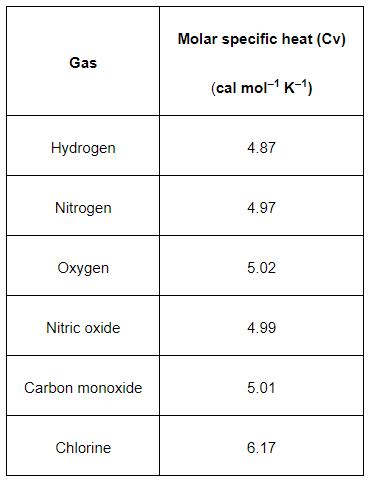The measured molar specific heats of these gases are markedly different from those for monatomic gases. Typically, molar specific heat of a monatomic gas is $2.92 \mathrm{cal} / \mathrm{mol} \mathrm{K}$. Explain this difference. What can you infer from the somewhat larger (than the rest) value for chlorine?

Solution. The gases listed in the given table are diatomic. Besides the translational degree of freedom, they have other degrees of freedom (modes of motion).
Heat must be supplied to increase the temperature of these gases. This increases the average energy of all the modes of motion. Hence, the molar specific heat of diatomic gases is more than that of monatomic gases.
If only rotational mode of motion is considered, then the molar specific heat of a diatomic
$\operatorname{gas}=\frac{5}{2} R$
$=\frac{5}{2} \times 1.98=4.95 \mathrm{cal} \mathrm{mol}^{-1} \mathrm{~K}^{-1}$
With the exception of chlorine, all the observations in the given table agree with $\left(\frac{5}{2} R\right)$. This is because at room temperature, chlorine also has vibrational modes of motion besides rotational and translational modes of motion.

Question.16: $Answer the following questions based on the$P-T$phase diagram of carbon dioxide: (a) At what temperature and pressure can the solid, liquid and vapour phases of$\mathrm{CO}_{2}$co-exist in equilibrium? (b) What is the effect of decrease of pressure on the fusion and boiling point of$\mathrm{CO}_{2} ?$(c) What are the critical temperature and pressure for$\mathrm{CO}_{2} ?$What is their significance? (d) Is$\mathrm{CO}_{2}$solid, liquid or gas at$(\mathrm{a})-70^{\circ} \mathrm{C}$under$1 \mathrm{~atm}$, (b)$-60^{\circ} \mathrm{C}$under$10 \mathrm{~atm}$, (c)$15^{\circ} \mathrm{C}$under$56 \mathrm{~atm} ?$Solution. (a) The P-T phase diagram for CO2 is shown in the following figure.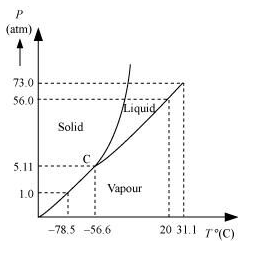$C$is the triple point of the$\mathrm{CO}_{2}$phase diagram. This means that at the temperature and pressure corresponding to this point (i.e., at$-56.6^{\circ} \mathrm{C}$and$\left.5.11 \mathrm{~atm}\right)$, the solid, liquid, and vaporous phases of$\mathrm{CO}_{2}$co-exist in equilibrium. (b) The fusion and boiling points of$\mathrm{CO}_{2}$decrease with a decrease in pressure.. (c) The critical temperature and critical pressure of$\mathrm{CO}_{2}$are$31.1^{\circ} \mathrm{C}$and 73 atm respectively. Even if it is compressed to a pressure greater than$73 \mathrm{~atm}, \mathrm{CO}_{2}$will not liquefy above the critical temperature. (d) It can be concluded from the$P-T$phase diagram of$\mathrm{CO}_{2}$that: (a)$\mathrm{CO}_{2}$is gaseous at$-70^{\circ} \mathrm{C}$, under 1 atm pressure (b)$\mathrm{CO}_{2}$is solid at$-60^{\circ} \mathrm{C}$, under 10 atm pressure (c)$\mathrm{CO}_{2}$is liquid at$15^{\circ} \mathrm{C}$, under 56 atm pressure Question.17: Answer the following questions based on the$P-T$phase diagram of$\mathrm{CO}_{2}$: Solution. (a)$\mathrm{CO}_{2}$at 1 atm pressure and temperature$-60^{\circ} \mathrm{C}$is compressed isothermally. Does it go through a liquid phase? (b) What happens when$\mathrm{CO}_{2}$at 4 atm pressure is cooled from room temperature at constant pressure? (c) Describe qualitatively the changes in a given mass of solid$\mathrm{CO}_{2}$at 10 atm pressure and temperature$-65^{\circ} \mathrm{C}$as it is heated up to room temperature at constant pressure. (d)$\mathrm{CO}_{2}$is heated to a temperature$70^{\circ} \mathrm{C}$and compressed isothermally. What changes in its properties do you expect to observe? Solution. (a) No (b) It condenses to solid directly. (c) The fusion and boiling points are given by the intersection point where this parallel line cuts the fusion and vaporisation curves. (d) It departs from ideal gas behaviour as pressure increases. Explanation: (a) The$P-T$phase diagram for$\mathrm{CO}_{2}$is shown in the following figure.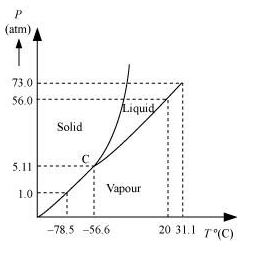At 1 atm pressure and at$-60^{\circ} \mathrm{C}, \mathrm{CO}_{2}$lies to the left of$-56.6^{\circ} \mathrm{C}$(triple point C). Hence, it lies in the region of vaporous and solid phases. Thus,$\mathrm{CO}_{2}$condenses into the solid state directly, without going through the liquid state. (b) At 4 atm pressure,$\mathrm{CO}_{2}$lies below$5.11$atm (triple point$\left.\mathrm{C}\right)$. Hence, it lies in the region of vaporous and solid phases. Thus, it condenses into the solid state directly, without passing through the liquid state. (c) When the temperature of a mass of solid$\mathrm{CO}_{2}$(at 10 atm pressure and at$\left.-65^{\circ} \mathrm{C}\right)$is increased, it changes to the liquid phase and then to the vaporous phase. It forms a line parallel to the temperature axis at$10 \mathrm{~atm}$. The fusion and boiling points are given by the intersection point where this parallel line cuts the fusion and vaporisation curves. (d) If$\mathrm{CO}_{2}$is heated to$70^{\circ} \mathrm{C}$and compressed isothermally, then it will not exhibit any transition to the liquid state. This is because$70^{\circ} \mathrm{C}$is higher than the critical temperature of$\mathrm{CO}_{2}$. It will remain in the vapour state, but will depart from its ideal behaviour as pressure increases. Question.18: A child running a temperature of$101^{\circ} \mathrm{F}$is given an antipyrin (i.e. a medicine that lowers fever) which causes an increase in the rate of evaporation of sweat from his body. If the is lost. The mass of the child is$30 \mathrm{~kg}$. The specific heat of human body is approximately the same as that of water, and latent heat of evaporation of water at that temperature is about 580 cal$\mathrm{g}^{-1}$Solution. Initial temperature of the body of the child,$T_{1}=101^{\circ} \mathrm{F}$Final temperature of the body of the child,$T_{2}=98^{\circ} \mathrm{F}$Change in temperature,$\Delta T=\left[(101-98) \times \frac{5}{9}\right]{ }^{\circ} \mathrm{C}$Time taken to reduce the temperature,$t=20 \mathrm{~min}$Mass of the child,$m=30 \mathrm{~kg}=30 \times 10^{3} \mathrm{~g}$Specific heat of the human body$=$Specific heat of water$=c=1000 \mathrm{cal} / \mathrm{kg} /{ }^{\circ} \mathrm{C}$Latent heat of evaporation of water,$L=580$cal$\mathrm{g}^{-1}$The heat lost by the child is given as:$\Delta \theta=m c \Delta T=30 \times 1000 \times(101-98) \times \frac{5}{9}=50000 \mathrm{cal}$Let$m_{1}$be the mass of the water evaporated from the child's body in$20 \mathrm{~min}$. Loss of heat through water is given by:$\Delta \theta=m_{1} L\therefore m_{1}=\frac{\Delta \theta}{L}=\frac{50000}{580}=86.2 \mathrm{~g}\therefore$Average rate of extra evaporation caused by the drug$=\frac{m_{1}}{t}=\frac{86.2}{200}=4.3 \mathrm{~g} / \mathrm{min}$Question.19: A 'thermacole' icebox is a cheap and efficient method for storing small quantities of cooked food in summer in particular. A cubical icebox of side$30 \mathrm{~cm}$has a thickness of$5.0 \mathrm{~cm}$.$4.0 \mathrm{~kg}$of ice is put in the box, estimate the amount of ice remaining after$6 \mathrm{~h}$. The outside temperature is$45^{\circ} \mathrm{C}$, and co-efficient of thermal conductivity of thermacole is$0.01 \mathrm{~J} \mathrm{~s}^{-1}\mathrm{m}^{-1} \mathrm{~K}^{-1}$. [Heat of fusion of water$=335 \times 10^{3} \mathrm{~J} \mathrm{~kg}^{-1}$] Solutioin. Side of the given cubical ice box,$s=30 \mathrm{~cm}=0.3 \mathrm{~m}$Thickness of the ice box,$I=5.0 \mathrm{~cm}=0.05 \mathrm{~m}$Mass of ice kept in the ice box,$m=4 \mathrm{~kg}$Time gap,$t=6 \mathrm{~h}=6 \times 60 \times 60 \mathrm{~s}$Outside temperature,$T=45^{\circ} \mathrm{C}$Coefficient of thermal conductivity of thermacole,$K=0.01 \mathrm{~J} \mathrm{~s}^{-1} \mathrm{~m}^{-1} \mathrm{~K}^{-1}$Heat of fusion of water,$L=335 \times 10^{3} \mathrm{~J} \mathrm{~kg}^{-1}$Let$m$' be the total amount of ice that melts in$6 \mathrm{~h}$. The amount of heat lost by the food:$\theta=\frac{K A(T-0) t}{l}$Where,$A=$Surface area of the box$=6 s^{2}=6 \times(0.3)^{2}=0.54 \mathrm{~m}^{3}\theta=\frac{0.01 \times 0.54 \times(45) \times 6 \times 60 \times 60}{0.05}=104976 \mathrm{~J}$But$\theta=m^{\prime} L\therefore m^{\prime}=\frac{\theta}{L}=\frac{104976}{335 \times 10^{3}}=0.313 \mathrm{~kg}$Mass of ice left$=4-0.313=3.687 \mathrm{~kg}$Hence, the amount of ice remaining after$6 \mathrm{~h}$is$3.687 \mathrm{~kg}$. Question. 20: A brass boiler has a base area of$0.15 \mathrm{~m}^{2}$and thickness$1.0 \mathrm{~cm}$. It boils water at the rate of$6.0 \mathrm{~kg} / \mathrm{min}$when placed on a gas stove. Estimate the temperature of the part of the flame in contact with the boiler. Thermal conductivity of brass$=109 \mathrm{~J} \mathrm{~s}^{-1} \mathrm{~m}^{-1} \mathrm{~K}^{-1} ;$Heat of vaporisation of water$=2256 \times 10^{3} \mathrm{~J} \mathrm{~kg}^{-1}$Solution. Base area of the boiler,$A=0.15 \mathrm{~m}^{2}$Thickness of the boiler,$I=1.0 \mathrm{~cm}=0.01 \mathrm{~m}$Boiling rate of water,$R=6.0 \mathrm{~kg} / \mathrm{min}$Mass,$m=6 \mathrm{~kg}$Time,$t=1 \min =60 \mathrm{~s}$Thermal conductivity of brass,$K=109 \mathrm{~J} \mathrm{~s}^{-1} \mathrm{~m}^{-1} \mathrm{~K}^{-1}$Heat of vaporisation,$L=2256 \times 10^{3} \mathrm{~J} \mathrm{~kg}^{-1}$The amount of heat flowing into water through the brass base of the boiler is given by:$\theta=\frac{K A\left(T_{1}-T_{2}\right) t}{l}$$\ldots(i) Where, T_{1}= Temperature of the flame in contact with the boiler T_{2}= Boiling point of water =100^{\circ} \mathrm{C} Heat required for boiling the water: \theta=m L \ldots(i i) Equating equations (i) and (ii), we get: \therefore m L=\frac{K A\left(T_{1}-T_{2}\right) t}{l} T_{1}-T_{2}=\frac{m L l}{K A t} =\frac{6 \times 2256 \times 10^{3} \times 0.01}{109 \times 0.15 \times 60} =137.98^{\circ} \mathrm{C} Therefore, the temperature of the part of the flame in contact with the boiler is 237.98^{\circ} \mathrm{C}. Question 21: Explain why: (a) a body with large reflectivity is a poor emitter (b) a brass tumbler feels much colder than a wooden tray on a chilly day. (c) an optical pyrometer (for measuring high temperatures) calibrated for an ideal black body radiation gives too low a value for the temperature of a red-hot iron piece in the open, but gives a correct value for the temperature when the same piece is in the furnace (d) the earth without its atmosphere would be inhospitably cold (e) heating systems based on circulation of steam are more efficient in warming a building than those based on circulation of hot water Solution. (a) A body with a large reflectivity is a poor absorber of light radiations. A poor absorber will in turn be a poor emitter of radiations. Hence, a body with a large reflectivity is a poor emitter (a) A body with a large reflectivity is a poor absorber of light radiations. A poor absorber will in turn be a poor emitter of radiations. Hence, a body with a large reflectivity is a poor emitter. (b) Brass is a good conductor of heat. When one touches a brass tumbler, heat is conducted from the body to the brass tumbler easily. Hence, the temperature of the body reduces to a lower value and one feels cooler. Wood is a poor conductor of heat. When one touches a wooden tray, very little heat is conducted from the body to the wooden tray. Hence, there is only a negligible drop in the temperature of the body and one does not feel cool. Thus, a brass tumbler feels colder than a wooden tray on a chilly day. (c) An optical pyrometer calibrated for an ideal black body radiation gives too low a value for temperature of a red hot iron piece kept in the open. Black body radiation equation is given by: E=\sigma\left(T^{4}-T_{0}^{4}\right) Where, E= Energy radiation T= Temperature of optical pyrometer T_{0}= Temperature of open space \sigma= Constant Hence, an increase in the temperature of open space reduces the radiation energy. When the same piece of iron is placed in a furnace, the radiation energy, E=\sigma T^{4} (d) Without its atmosphere, earth would be inhospitably cold. In the absence of atmospheric gases, no extra heat will be trapped. All the heat would be radiated back from earth’s surface. (e) A heating system based on the circulation of steam is more efficient in warming a building than that based on the circulation of hot water. This is because steam contains surplus heat in the form of latent heat (540 cal/g). Question 22: A body cools from 80^{\circ} \mathrm{C} to 50{ }^{\circ} \mathrm{C} in 5 minutes. Calculate the time it takes to cool from 60^{\circ} \mathrm{C} to 30^{\circ} \mathrm{C}. The temperature of the surroundings is 20^{\circ} \mathrm{C} Solution. According to Newton's law of cooling, we have: -\frac{d T}{d t}=K\left(T-T_{0}\right) \frac{d T}{K\left(T-T_{0}\right)}=-K d t$$\ldots(i)$Where, Temperature of the body$=T$Temperature of the surroundings$=T_{0}=20^{\circ} \mathrm{C}K$is a constant Temperature of the body falls from$80^{\circ} \mathrm{C}$to$50^{\circ} \mathrm{C}$in time,$t=5 \mathrm{~min}=300 \mathrm{~s}$Integrating equation$(i)$, we get:$\int_{50}^{80} \frac{d T}{K\left(T-T_{0}\right)}=-\int_{0}^{300} K d t\left[\log _{e}\left(T-T_{0}\right)\right]_{50}^{80}=-K[t]_{0}^{300}\frac{2.3026}{K} \log _{10} \frac{80-20}{50-20}=-300\frac{2.3026}{K} \log _{10} 2=-300\frac{-2.3026}{300} \log _{10} 2=K$$\ldots(i i) The temperature of the body falls from 60^{\circ} \mathrm{C} to 30^{\circ} \mathrm{C} in time =t Hence, we get: \frac{2.3026}{K} \log _{10} \frac{60-20}{30-20}=-t \frac{-2.3026}{t} \log _{10} 4=K$$\ldots(i i i)$Equating equations (ii) and (iii), we get:$\frac{-2.3026}{t^{\prime}} \log _{10} 4=\frac{-2.3026}{300} \log _{10} 2\therefore t=300 \times 2=600 \mathrm{~s}=10 \mathrm{~min}$Therefore, the time taken to cool the body from$60^{\circ} \mathrm{C}$to$30^{\circ} \mathrm{C}\$ is 10 minutes.